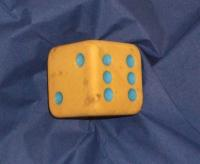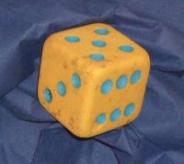You may also likeKnock-out

Before a knockout tournament with 2^n players I pick two players. What is the probability that they have to play against each other at some point in the tournament?Squash

If the score is 8-8 do I have more chance of winning if the winner is the first to reach 9 points or the first to reach 10 points?Snooker

A player has probability 0.4 of winning a single game. What is his probability of winning a 'best of 15 games' tournament?

Dicey Decisions

Age 16 to 18Challenge Level

This problem follows on from Troublesome Dice.

Jenny and her family often roll a die to decide who gets to eat the last slice of pie, but when they roll it, the die often seems to land "edge-up" or "corner-up" on a napkin, so they roll again.

One day, Jenny suggests that instead of rolling again, when the die lands corner-up or edge-up, they simply add the visible faces together:What assumptions would you need to make to come up with a fair way of determining who gets the last slice of pie?

Here are some questions you might like to consider:

Suppose the die lands face-up with probability $\frac {1}{2}$, edge-up with probability $\frac{1}{3}$ and corner-up with probability $\frac{1}{6}$. How could you allocate totals to three people to give each of them an equal chance of getting the last slice of pie?

Suppose the die lands face-up with probability $p$, edge-up with probability $q$, and corner-up with probability $r = 1-(p+q)$. Are there any family sizes for which you can always allocate totals fairly, regardless of the values of $p$, $q$ and $r$?

The relative frequency of face-up, edge-up and corner-up scores for the standard 6-sided die are distributed symmetrically. Can you explain why?

This problem is based on an idea shared by Paul Stephenson.